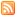25yicms利用ASP.NET(C#)+MSSQL技术全力打造功能最强大的营销型企业网站管理系统,企业做网站系统,做网站软件，提供div+css企业网站模板。RSS

## C#时间比较和时间差值计算,某时间减多少天(时,秒)的计算

1.
string timea="12:13";
string timeb="18:13";
DateTime dt1=Convert.ToDateTime(timea);
DateTime dt2=Convert.ToDateTime(timeb);
if(dt1>dt2)  {  ……操作代码  }
2.
string timea="12:13";
string timeb="18:13";
DateTime dt1=Convert.ToDateTime(timea);
DateTime dt2=Convert.ToDateTime(timeb);
int n=dt1.CompareTo(dt2);
if(n>0)  {  ……操作代码  }

C#时间差值计算一般是使用时间间隔函数TimeSpan和其属性Subtract:从中减去另一个TimeSpan值。

TimeSpan ts1=newTimeSpan(DateTime1.Ticks);
TimeSpan ts2=newTimeSpan(DateTime2.Ticks);
TimeSpan ts=ts1.Subtract(ts2).Duration();
dateDiff=ts.Days.ToString()+"天" +ts.Hours.ToString()+"小时" +ts.Minutes.ToString()+"分钟" +ts.Seconds.ToString()+"秒";

TimeSpan ts=new TimeSpan(50,0,0,0);
DateTimedt2=DateTime.Now.Subtract(ts);